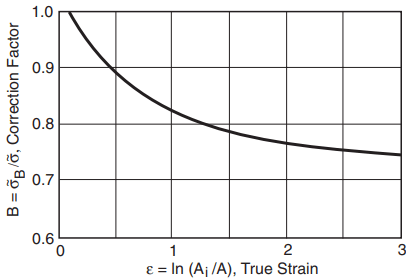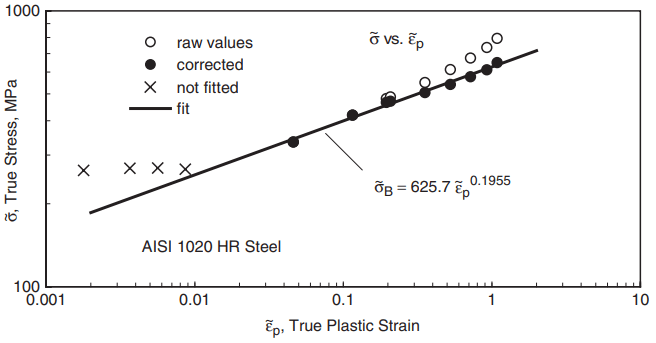In analyzing the results of tension tests, and in certain other situations, it is useful to work with true stresses and strains. Note that engineering stress and strain are most appropriate for small strains where the changes in specimen dimensions are small. True stresses and strains differ in that finite changes in area and length are specifically considered. For a ductile material, plotting true stress and strain from a tension test gives a curve that differs markedly from the engineering stress–strain curve. An example is shown in Fig. 1.Figure 1 Engineering and true stress–strain curves from a tension test on hot-rolled AISI 1020 steel.

## Definitions of True Stress and Strain

True stress is simply the axial force P divided by the current cross-sectional area A, rather than the original area Ai. Hence, given A, true stress σ˜ may be calculated from force P or from engineering stress σ:

Since the area A decreases as a tension test proceeds, true stresses increasingly rise above the corresponding engineering stresses. Also, there is no drop in stress beyond an ultimate point, which is expected, as this behavior in the engineering stress–strain curve is due to the rapid decrease in cross-sectional area during necking. These trends are evident in Fig. 1.

For true strain, let the length change be measured in small increments, ΔL1, ΔL2, ΔL3, etc., and let the new gage length, L1, L2, L3, etc., be used to compute the strain for each increment. The total strain is thus

where ΔL is the sum of these ΔLj . If the ΔLj are assumed to be infinitesimal—that is, if ΔL is measured in very small steps—the preceding summation is equivalent to an integral that defines true strain:

Here, L = Li + ΔL is the final length. Note that ε = ΔL/Li is the engineering strain, leading to the following relationship between ε and ε˜:

## Constant Volume Assumption

For materials that behave in a ductile manner, once the strains have increased substantially beyond the yield region, most of the strain that has accumulated is inelastic strain. Since neither plastic strain nor creep strain contributes to volume change, the volume change in a tension test is limited to the small amount associated with elastic strain. Thus, it is reasonable to approximate the volume as constant:

Substitution into Eqs. 4.12(b) and 4.14 then gives two additional equations relating true and engineering stress and strain:

For members with round cross sections of original diameter di and final diameter d, the last equation may be used in the form

It should be remembered that Eqs. 4.17 through 4.20 depend on the constant volume assumption and may be inaccurate unless the inelastic (plastic plus creep) strain is large compared with the elastic strain.

True strains from Eq. 4 are somewhat smaller than the corresponding engineering strains. But once necking starts and Eq. 9 is employed with the rapidly decreasing values of A, the true strain may increase substantially beyond the engineering strain, as seen in Fig. 1.

## Limitations on True Stress–Strain Equations

The ranges of applicability of the various equations for calculating engineering and true stresses and strains are summarized by Fig. 2. First, note that engineering stress and strain may always be determined from their definitions. True stress may always be obtained from Eq. 1 if areas are directly measured, as from diameters in round cross sections.Figure 2 Use and limitations of various equations for stresses and strains from a tension test.

Once necking starts at the engineering ultimate stress point, the engineering strain becomes merely an average over a region of non-uniform deformation. Hence, it does not represent the maximum strain and becomes unsuitable for calculating true stresses and strains.

This situation requires that Eqs. 4 and 7 not be used beyond the ultimate point. Beyond this, true stresses and strains can be calculated only if the varying minimum cross-sectional area in the necked region is measured, as by measuring diameters for round specimens. Hence, only Eqs. 1 and 9 are available beyond the ultimate point where necking starts.

In addition, Eq. 7 is limited by the constant volume assumption. Hence, this conversion to true stress is inaccurate at small strains, such as those below and around the yield stress.

An arbitrary lower limit of twice the strain that accompanies the offset yield strength, 2εo, is suggested in Fig. 2. Below this limit, the difference between true and engineering stress is generally so small that it can be neglected, so that no conversion is needed. A similar limitation is encountered by Eqs. 8 and 9, which are otherwise valid at any strain.

## Bridgman Correction for Hoop Stress

A complication exists in interpreting tensile results near the end of a test where there is a large amount of necking. As pointed out by P. W. Bridgman in 1944, large amounts of necking result in a tensile hoop stress being generated around the circumference in the necked region. Thus, the state of stress is no longer uniaxial as assumed, and the behavior of the material is affected. In particular, the axial stress is increased above what it would otherwise be. This is caused by plastic deformation.Figure 3 Curve of [Bridgman 44] giving correction factors on true stress for the effect of hoop stress due to necking in steels.

A correction for steel can be made on the basis of the empirical curve developed by Bridgman, which is shown in Fig. 3. The curve is entered with the true strain based on area, and it gives a value of the correction factor, B, which is used as follows:

σ˜B = Bσ˜……(10)

Here, σ˜ is true stress simply computed from area by using Eq. 1, and σ˜B is the corrected value of true stress. Values of B for steels may be estimated from the following equation that closely approximates the curve of Fig. 3:

B = 0.0684x3 + 0.0461x2 − 0.205x + 0.825, where x = log10 ε˜ ( 0.12 ≤ ε˜ ≤ 3)……..(11)

The correction is not needed for ε˜ < 0.12. Note that a 10% correction (B = 0.9) corresponds to a true strain of  ε˜ = 0.44. By Eq. 9, this gives a ratio of initial to necked diameter of 1.25. Hence, fairly large strains must occur for the correction to be significant.

Similar correction curves are not generally available for other metals, but a correction can still be done if the radius of the neck profile is measured. The one for steel should not be applied to other metals except as a rough approximation.

## True Stress–Strain Curves

For true stress–strain curves of metals in the region beyond yielding, the stress versus plastic strain behavior often fits a power relationship:

If stress versus plastic strain is plotted on log–log coordinates, this equation gives a straight line.
The slope is n, which is called the strain hardening exponent. The quantity H, which is called
the strength coefficient, is the intercept at ε˜p = 1. At large strains during the advanced stages
of necking, Eq. 12 should be used with true stresses that have been corrected by means of the
Bridgman factor:

A log–log plot of true stress versus true plastic strain for the test on AISI 1020 steel is shown in Fig. 4. Note that the σ˜ versus ε˜ p data curve upward at the higher strains, but the σ˜B versus ε˜ p (corrected) data do lie along an apparent straight line trend, to which a power relationship of the form of Eq. 13 has been fitted as shown.Figure 4 Log–log plot of true stress versus true plastic strain for AISI 1020 hot-rolled steel.

Since the total strain is the sum of its elastic and plastic parts, Eq. 13 can be solved for plastic strain, and this can be added to the elastic strain, ε˜e = ˜σB/E, to obtain a relationship between true stress and the total true strain ε˜:

This form is called the Ramberg–Osgood relationship.

Using the constants for the example AISI 1020 steel to plot Eq. 14, we obtain the dashed curve in Fig. 1. This is seen to represent the corrected data very well over all strains that are beyond the anomalous upper/lower yield region of this material. Where the stress–strain curve exhibits a smooth, gradual yielding behavior, Eq. 14 may provide a good fit for all strains.

For some materials, Eq. 14 does not fit very well, and some cases of this type can be handled by two power laws that fit different portions of the stress–strain curve.

If cross-sectional area (diameter) measurements are not available for a tension test, then Eq. 14 may still be employed, but the fit is restricted to data that are not beyond the engineering ultimate stress point.

The Bridgman correction then becomes unnecessary, so that Eq. 12 applies, and σ˜B in Eq. 14 is replaced by the uncorrected σ˜. Also, since the ultimate point is not exceeded, σ˜ and ε˜ may be calculated simply from Eqs. 7 and 4.

## True Stress–Strain Properties

Additional materials properties obtained from tension tests may be defined on the basis of true stress and strain. The true fracture strength, σf˜, is obtained simply from the load at fracture and the final area, or from the engineering stress at fracture:

Since the Bridgman correction is generally needed, the value obtained should be converted to σf˜B by using Eq. 10, but note the limitation of Eq. 11 to steels.

The true fracture strain, εf˜, may be obtained from the final area or from the percent reduction in area:

The second equation follows readily from the first. Note that εf˜ cannot be computed if only the engineering strain at fracture (percent elongation) is available. The true toughness, uf˜, is the area under the true stress–strain curve up to fracture. Assume that the material is quite ductile, so that the elastic strains are small, compared with the plastic strains, over most of the stress–strain curve. Hence, let the plastic and total strains be taken as equivalent, ε˜p ≈ ε˜, and consequently replace Eq. 13 with σ˜B = Hε˜n. On this basis, the true toughness is

The various engineering and true stress–strain properties from tension tests are summarized by the categorized listing of Table 1.

Note that the engineering fracture strain εf and the percent elongation are only different ways of stating the same quantity. Also, the %R A and ε˜f can each be calculated from the other by using Eq. 16(b).

The strength coefficient H determines the magnitude of the true stress in the large strain region of the stress–strain curve, so it is included as a measure of strength. The strain hardening exponent n is a measure of the rate of strain hardening for the true stress–strain curve.

For engineering metals, values above n = 0.2 are considered relatively high, and those below 0.1 are considered relatively low. True stress–strain properties are listed for several engineering metals in Table 2.